Latest Banking jobs   »   Quantitative Aptitude Quiz For RRB PO...   »   Quantitative Aptitude Quiz For RRB PO...

# Quantitative Aptitude Quiz For RRB PO Mains 2021- 9th September

Q1. Total distance between Delhi to Lucknow is 480 km. A train starts running with an average speed of 60 km/hr from Delhi to Lucknow while another train starts Journey after 120 minutes of first train and reaches Lucknow 30 minutes before first train. If first train stops for 5 minutes on each station and second train did not stop at any station ,then find the ratio between speed of first train to speed of second train given that total number of station between Delhi and Lucknow are nine.?
(a) 25 : 33
(b) 25 : 32
(c) 35 : 25
(d) 25 : 31
(e) 21 : 31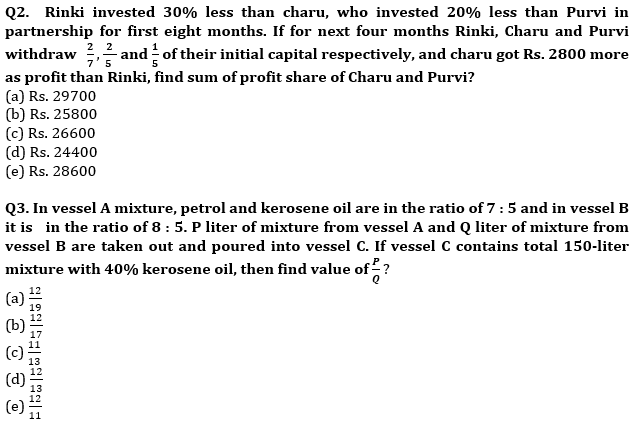Q4. Rakesh lent Rs. P to Rahul on an agreement that for first two year, interest will be calculated on SI at 15% per annum and for next two years interest will be calculated on C.I at 5% additional rate. If Rahul paid total amount of Rs. 17971.2 at the end of four year, Find the amount borrowed by Rahul?
(a) 9000 Rs.
(b) 9800 Rs.
(c) 9900 Rs.
(d) 9600 Rs.
(e) 9200 Rs.

Q5. Marked price of two articles A and B are in the ratio of 7 : 9, at the time of selling shopkeeper gives discount of d% on article A and (d + 5)% on article B and made a profit of 25% on each article, if cost price of article A and B are in ratio 112 : 135. Then find percent of discount given by shopkeeper on both articles?
(a) 12.5%, 17.5%
(b) 25%, 30%
(c) 10%, 15%
(d) 15 %, 20%
(e) 20%, 25%

Q6. A train M running at the speed of 108 km/hr crosses a man running at 12 km/hr in opposite direction of train in 12 second and crosses a platform in 32 sec. If a train N is Standing on same platform and length of platform is 140 meters more than length of train N. Find in what time train N will cross train M running in same direction, if train N passes a pole in 12 second?
(a) 168 sec
(b) 164 sec
(c) 154 sec
(d) 186 sec
(e) 172 sec

Q7. Ritu invested her total saving in three different FD schemes A, B and C in the ratio of 5 : 4 : 6 on CI for two years at the rate of 10%, 15% and 20% respectively. If interest is calculated annually and interest from scheme B is Rs. 744 more than interest from scheme A then, find difference between interest received from scheme C and scheme B by Ritu?
(a) Rs. 4185
(b) Rs. 4175
(c) Rs. 3840
(d) Rs. 4580
(e) Rs. 3250

Q8. A boat goes certain distance downstream and then return 3/4th of the distance upstream. It takes 3/2 of the time in upstream than in downstream. If boat increases its speed by 33⅓% and cover a distance of 60 km in downstream and then return upstream in 16 hours, find increased speed of boat?
(a) 6 km/hr
(b) 14 km/hr
(c) 16 km/hr
(d) 12 km/hr
(e) 8 km/hr

Q9. In a house, there is a semicircular corridor, a circular hall and a circular garden. Sum of perimeter of corridor and hall is 102 mi. radius of hall is 50% more than radius of corridor then find area of circular garden, given radius of garden is 300% more than sum of radius of corridor and radius of hall together.
(a) 6300π sq.m
(b) 5400π sq.m
(c) 5700π sq.m
(d) 4200π sq.m
(e) 4900π sq.m

Q10. The marked price of a bike in two stores A and B is same. In store A bike is available at 30% discount and in store B same bike is available at two successive discounts of d% and 8%. A man bought bike from store A at Rs. 49000. If man would have paid Rs. 7672 more, he could have bought the same bike from store B. Find the discount ‘d’ allowed by store B on bike?
(a) 8%
(b) 10%
(c) 12%
(d) 16%
(e) 15%

Solutions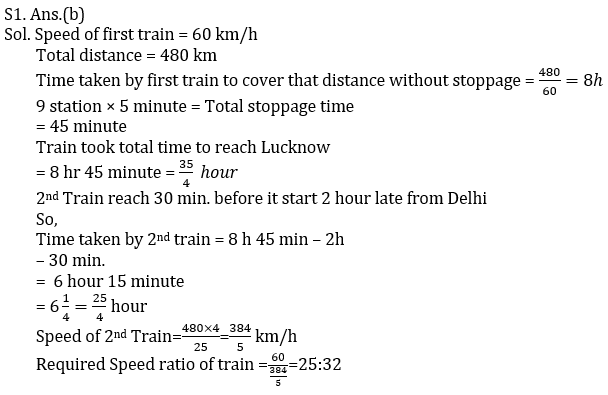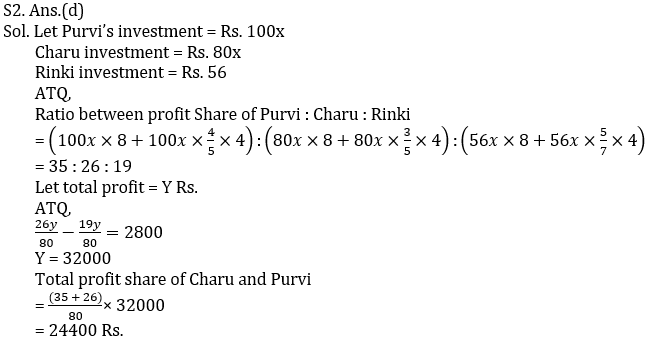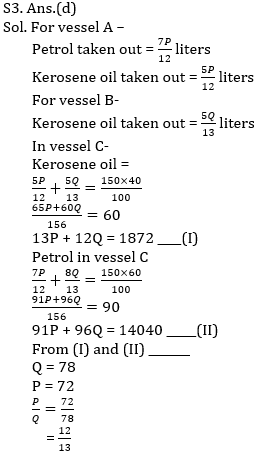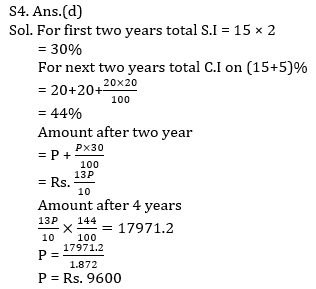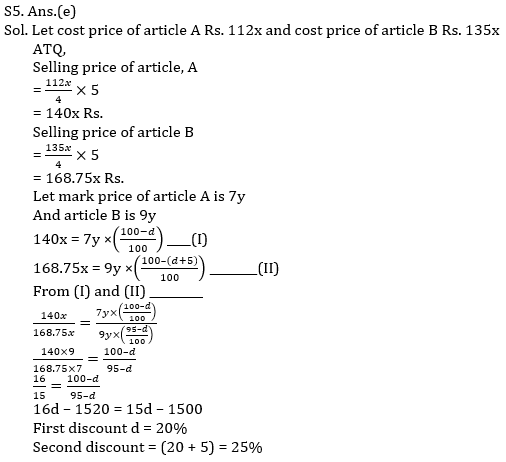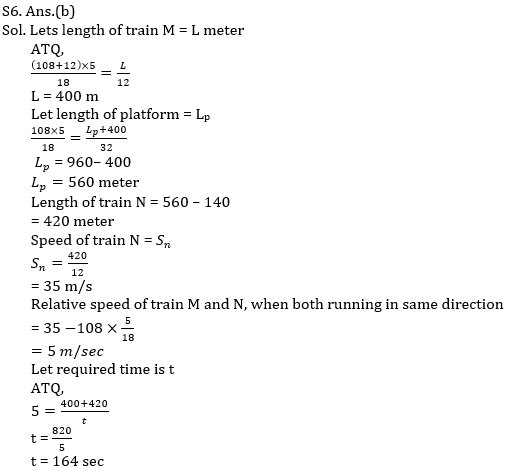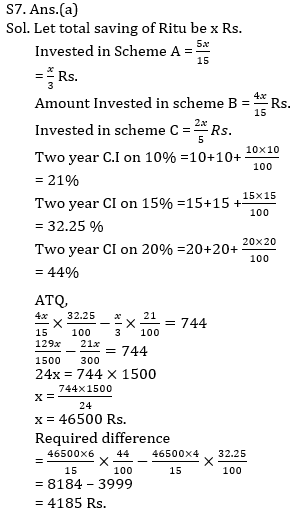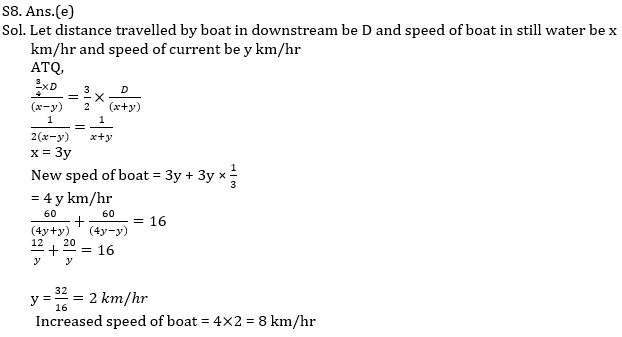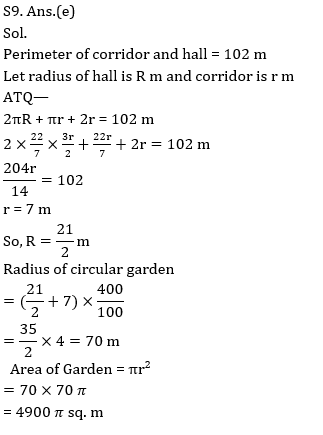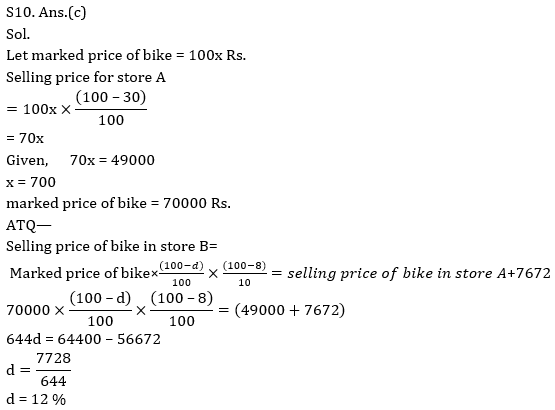Click Here to Register for Bank Exams 2021 Preparation Material#### Congratulations!Download Hindu Review of October 2021: Free PDF